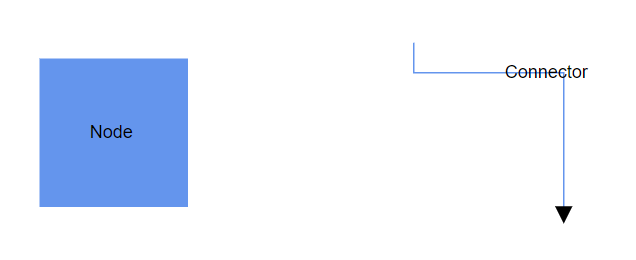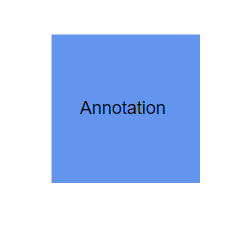# Annotation in Blazor Diagram Component

28 Dec 20219 minutes to read

The Annotation is a block of text that can be displayed over a node or connector and it is used to textually represent an object with a string that can be edited at run time. Multiple annotations can be added to a node or connector.

## Create annotations

An annotation can be added to a node or connector by defining the annotation object and adding that to the annotation collection of the node or connector. The Content property of annotation defines the text to be displayed. The following code explains how to create an annotation.

``````@using Syncfusion.Blazor.Diagram

<SfDiagramComponent Height="600px" Nodes="@nodes" Connectors="@connectors" />

@code
{
// Defines diagram's connector collection.
DiagramObjectCollection<Connector> connectors;

// Defines diagram's node collection.
DiagramObjectCollection<Node> nodes;

protected override void OnInitialized()
{
nodes = new DiagramObjectCollection<Node>();
Node node = new Node()
{
Width = 100,
Height = 100,
OffsetX = 100,
OffsetY = 100,
Style = new ShapeStyle() { Fill = "#6495ED", StrokeColor = "white" },
Annotations = new DiagramObjectCollection<ShapeAnnotation>()
{
// Annotation is created and stored in Annotations collection of Node.
new ShapeAnnotation { Content = "Node" }
}
};
connectors = new DiagramObjectCollection<Connector>();
Connector connector = new Connector()
{
SourcePoint = new DiagramPoint() { X = 300, Y = 40 },
TargetPoint = new DiagramPoint() { X = 400, Y = 160 },
Type = ConnectorSegmentType.Orthogonal,
Style = new TextStyle() { StrokeColor = "#6495ED" },
Annotations = new DiagramObjectCollection<PathAnnotation>()
{
// Annotation is created and stored in Annotations collection of Connector.
new PathAnnotation { Content = "Connector" }
}
};
}
}``````• ID for each annotation should be unique and so it is further used to find the annotation at runtime and do any customization.
• By default, node’s annotation positioned in center point of the shape.
• By default, connector’s path annotation positioned in center point of its path.

You can add Annotation at runtime to the Annotations collection of the node/connector in the diagram component by using the `Add` method.

The following code explains how to add an annotation to a node at runtime by using `Add` method.

``````@using Syncfusion.Blazor.Diagram

<SfDiagramComponent Height="600px" @ref="@diagram" Nodes="@nodes">
</SfDiagramComponent>

@code
{
// Reference to diagram.
SfDiagramComponent diagram;

// Defines diagram's node collection.
DiagramObjectCollection<Node> nodes;

protected override void OnInitialized()
{
nodes = new DiagramObjectCollection<Node>();
Node node = new Node()
{
Width = 100,
Height = 100,
OffsetX = 100,
OffsetY = 100,
Style = new ShapeStyle() { Fill = "#6495ED", StrokeColor = "white" },
};
}

// Method to add annotation at runtime.
{
ShapeAnnotation annotation = new ShapeAnnotation { Content = "Annotation" };
}
}``````

Also, the annotations can be added at runtime by using the AddAsync method. The `await` operator suspends evaluation of the enclosing async method until the asynchronous operation represented by its operand completes.

The following code explains how to add an annotation to a node at runtime by using the `AddAsync` method.

``````//Method to add annotation at runtime.
{
ShapeAnnotation annotation = new ShapeAnnotation { Content = "Annotation" };
}``````## Remove annotations

A collection of annotations can be removed from the node by using the `RemoveAt` method. The following code explains how to remove an annotation from a node.

``````@using Syncfusion.Blazor.Diagram

<input value="Removelabel" type="button" @onclick="@RemoveLabel" name="Removelabel" />

<SfDiagramComponent Height="600px" @ref="@diagram" Nodes="@nodes" />

@code
{
// Reference to diagram.
SfDiagramComponent diagram;

// Defines diagram's node collection.
DiagramObjectCollection<Node> nodes;

protected override void OnInitialized()
{
nodes = new DiagramObjectCollection<Node>();
Node node = new Node()
{
Width = 100,
Height = 100,
OffsetX = 100,
OffsetY = 100,
Style = new ShapeStyle() { Fill = "#6BA5D7", StrokeColor = "white" },
};
node.Annotations = new DiagramObjectCollection<ShapeAnnotation>()
{
new ShapeAnnotation {ID = "label", Content = "Annotation" },
};
}

// Method to remove annotation at runtime.
public void RemoveLabel()
{
(diagram.Nodes.Annotations as DiagramObjectCollection<ShapeAnnotation>).RemoveAt(0);
}
}``````

Also, a collection of annotations can be removed from the node by using the `Remove` method.

``````    // Method to remove annotation at runtime using Remove method.
public void RemoveLabel()
{
ShapeAnnotation annotation = (diagram.Nodes.Annotations) as ShapeAnnotation;
(diagram.Nodes.Annotations as DiagramObjectCollection<ShapeAnnotation>).Remove(annotation);
}``````
• You can delete multiple annotations from node to pass the collection of annotation objects as argument.
• The `Add`, `Remove`, and `RemoveAt` methods are applicable for connectors too.

## Update annotations at runtime

You can get the annotation directly from the node’s annotations collection property and you can change any annotation properties at runtime.

The following code sample shows how the annotation of the node changed at runtime.

``````@using Syncfusion.Blazor.Diagram

<input value="Updatelabel" type="button" @onclick="@UpdateLabel" name="Updatelabel" />

<SfDiagramComponent Height="600px" @ref="@diagram" Nodes="@nodes" />

@code
{
// Reference to diagram.
SfDiagramComponent diagram;

// Defines diagram's node collection.
DiagramObjectCollection<Node> nodes;

protected override void OnInitialized()
{
nodes = new DiagramObjectCollection<Node>();
Node node = new Node()
{
Width = 100,
Height = 100,
OffsetX = 100,
Annotations = new DiagramObjectCollection<ShapeAnnotation>()
{
new ShapeAnnotation { Content = "Node" }
},
OffsetY = 100,
Style = new ShapeStyle() { Fill = "#6495ED", StrokeColor = "white" },
};